# MCQ in Calculus Part 4 | ECE Board Exam

(Last Updated On: February 6, 2020)This is the Multiple Choice Questions Part 4 of the Series in Calculus topic in Engineering Mathematics. In Preparation for the ECE Board Exam make sure to expose yourself and familiarize in each and every questions compiled here taken from various sources including but not limited to past Board Examination Questions in Engineering Mathematics, Mathematics Books, Journals and other Mathematics References.

### Multiple Choice Questions Topic Outline

• MCQs in Complex Variables | MCQs in Derivatives and Applications | MCQs in Integration and Applications | MCQs in Transcendental Functions | MCQs in Partial Derivatives | MCQs in Indeterminate forms | MCQs in Multiple Integrals | MCQs in Differential Equations | MCQs in Maxima/Minima and Time Rates

### Continue Practice Exam Test Questions Part IV of the Series

Choose the letter of the best answer in each questions.

151. An object falls from rest in a medium offering a resistance. The velocity of the object before the object reaches the ground is given by the differential equation dV/dt + V/10 = 32 ft/sec. What is the velocity of the object one second after it falls?

• A. 40.54
• B. 38.65
• C. 30.45
• D. 34.12

152. In a tank are 100 liters of brine containing 50 kg. total of dissolved salt. Pure water is allowed to run into the tank at the rate of 3 liters a minute. Brine runs out of the tank at the rate of 2 liters a minute. The instantaneous concentration in the tank is kept uniform by stirring. How much salt is in the tank at the end of one hour?

• A. 15.45 kg.
• B. 19.53 kg.
• C. 12.62 kg.
• D. 20.62 kg.

153. A tank initially holds 100 gallons of salt solution in which 50 lbs of salt has been dissolved. A pipe fills the tank with brine at the rate of 3 gpm, containing 2 lbs of dissolved salt per gallon. Assuming that the mixture is kept uniform by stirring, a drain pipe draws out of the tank the mixture at 2 gpm. Find the amount of salt in the tank at the end of 30 minutes.

• A. 171.24 lbs
• B. 124.11 lbs
• C. 143.25 lbs
• D. 105.12 lbs

154. If the nominal interest rate is 3%, how much is P5,000 worth in 10 years in a continuously compounded account?

• A. P5,750
• B. P6,750
• C. P7,500
• D. P6,350

155. A nominal interest of 3V compounded continuously is given on the account. What is the accumulated amount of P10,000 after 10 years?

• A. P13,620.10
• B. P13,500.10
• C. P13,650.20
• D. P13,498.60

156. Simplify the expression i^1997 + i^1999, where i is an imaginary number.

• A. 0
• B. –i
• C. 1 + i
• D. 1 – i

157. Simplify: i^29 + i^21 + i

• A. 3i
• B. 1 – i
• C. 1 + i
• D. 2i

158. Write in the form a + bi the expression i^3217 – i^427 + i^18

• A. 2i +1
• B. –i +1
• C. 2i – 1
• D. 1 + i

159. The expression 3 + 4i is a complex number. Compute its absolute value.

• A. 4
• B. 5
• C. 6
• D. 7

160. Write the polar form of the vector 3 + j4.

• A. 6 cis 53.1°
• B. 10 cis 53.1°
• C. 5 cis 53.1°
• D. 8 cis 53.1°

161. Evaluate the value of sqrt(-10) x sqrt(-7).

• A. i
• B. –sqrt(70)
• C. sqrt(70)
• D. sqrt(17)

162. Simplify (3 – i)^2 – 7(3 – i) + 10

• A. – (3 + i)
• B. 3 + i
• C. 3 – i
• D. – (3 – i)

163. If A = 40 e^(j120°), B = 2 cis -40°, C = 26.46 + j0, solve for A + B + C.

• A. 27.7 cis 45°
• B. 35.1 cis 45°
• C. 30.8 cis 45°
• D. 33.4 cis 45°

164. What is 4i cube times 2i square?

• A. -8i
• B. 8i
• C. -8
• D. -8i^2

165. What is the simplified complex expression of (4.33 + j2.5) square?

• A. 12.5 + j21.65
• B. 20 + j20
• C. 15 + j20
• D. 21.65 + j12.5

166. Find the value of (1 + i)^5, where I is an imaginary number.

• A. 1 – i
• B. -4(1 + i)
• C. 1 + i
• D. 4(1 + i)

167. Find the principal 5th root of [ 50(cos 150° + jsin 150°) ].

• A. 1.9 + j1.1
• B. 3.26 – j2.1
• C. 2.87 + j2.1
• D. 2.25 – j1.2

168. What is the quotient when 4 + 8i is divided by i^3?

• A. 8 – 4i
• B. 8 + 4i
• C. -8 + 4i
• D. -8 – 4i

169. If A = -2 – 3i, and B = 3 + 4i, what is A/B?

• A. (18 – i)/25
• B. (-18 – i)/25
• C. (-18 + i)/25
• D. (18 + i)/25

170. Rationalize (4 + 3i) / (2 – i).

• A. 1 + 2i
• B. (11 + 10i)/5
• C. (5 + 2i)/5
• D. 2 + 2i

171. Simplify [(2 + 3i)(5 – i)] / [(3 – 2i)^2].

• A. (221 – 91i)/169
• B. (21 + 52i)/13
• C. (-7 + 17i)/13
• D. (-90 + 220i)/169

172. What is the simplified expression of the complex number (6 + j2.5)/(3 + j4)?

• A. – 0.32 + j0.66
• B. 1.12 – j0.66
• C. 0.32 – j0.66
• D. – 1.75 + j1.03

173. Perform the operation: 4 (cos 60° + i sin 60°) divided by 2 (cos 30° + i sin 30°) in rectangular coordinates.

• A. square root of 3 – 2i
• B. square root of 3 – i
• C. square root of 3 + i
• D. square root of 3 + 2i

174. Find the quotient of (50 + j35)/(8 + j5).

• A. 6.47 cis 3°
• B. 4.47 cis 3°
• C. 7.47 cis 30°
• D. 2.47 cis 53°

175. Three vectors A, B and C are related as follows: A/B = 2 at 180°, A + C = -5 + j15, C = conjugate of B. Find A.

• A. 5 – j5
• B. -10 + j10
• C. 10 – j10
• D. 15 + j15

176. Evaluate cosh(jπ/4).

• A. 0.707
• B. 1.41 + j0.866
• C. 0.5 + j0.707
• D. j0.707

177. Evaluate tanh(jπ/3).

• A. 0.5 + j1.732
• B. j0.866
• C. j1.732
• D. 0.5 + j0.866

178. Evaluate in (2 + j3).

• A. 1.34 + j0.32
• B. 2.54 + j0.866
• C. 2.23 + j0.21
• D. 1.28 + j0.98

179. Evaluate the terms of a Fourier series 2 e^(j10πt) + e^(- j10πt) at t = 1.

• A. 2 + j
• B. 2
• C. 4
• D. 2 + j2

180. Given the following series:

sin x = x – x^3/3! + x^5/5! + …

cos x 1 – x^2/2! + x^4/4! + …

e^x = 1 + x + x^2/2! + x^3/3! + …

What relation can you draw from these series?

• A. e^x = cos x + sin x
• B. e^(ix) = cos x + i sin x
• C. e^(ix) = I cos x + sin x
• D. ie^x = i cos x + i sin x

181. One term of a Fourier series in cosine form is 10 cos 40πt. Write it in exponential form.

• A. 5 e^(j40πt)
• B. 5 e^(j40πt) + 5 e^(-j40πt)
• C. 10 e^(-j40πt)
• D. 10 e^(j40πt)

182. Evaluate the determinant: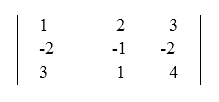• A. 4
• B. 2
• C. 5
• D. 0

183. Evaluate the determinant: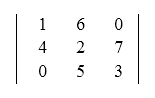• A. 110
• B. -101
• C. 101
• D. -110

184. Evaluate the determinant: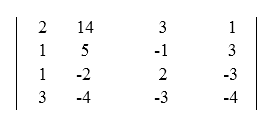• A. 489
• B. 389
• C. 326
• D. 452

185. Compute the value of x by determinant.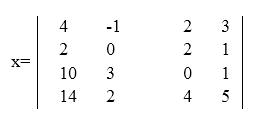• A. -32
• B. -28
• C. 16
• D. 52

186. Given the equations:

x + y + z = 2

3x – y – 2z = 4

5x – 2y + 3z = -7

Solve for y by determinants.

• A. 1
• B. -2
• C. 3
• D. 0

187. Solve the equations by Cramer’s Rule:

2x – y + 3z = -3

3x + 3y – z = 10

-x – y + z = -4

• A. (2, 1, -1)
• B. (2, -1, -1)
• C. (1, 2, -1)
• D. (-1, -2, 1)

188. If,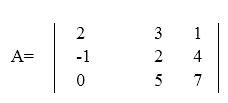What is the cofactor of the second row, third column element?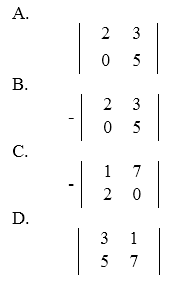189. If,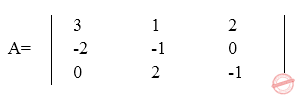What is the cofactor with the first row, second column element?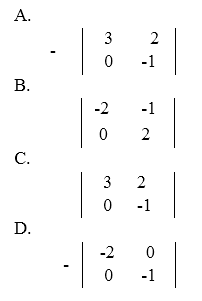190. If a 3 x 3 matrix and its inverse are multiplied together, write the product.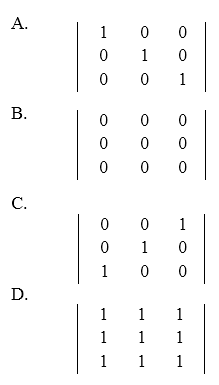191. If matrix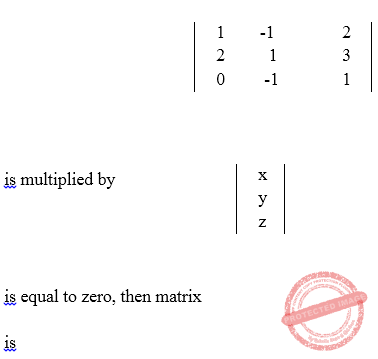• A. 3
• B. 1
• C. 0
• D. -2

192. Given the matrix equation, solve for x and y.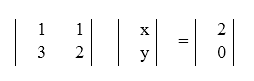• A. -4, 6
• B. -4, 2
• C. -41 -2
• D. -4, -6

193. If matrix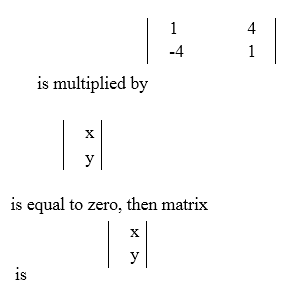• A. 8
• B. 1
• C. -4
• D. 0

194. If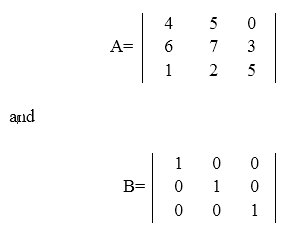What is A times B equal to?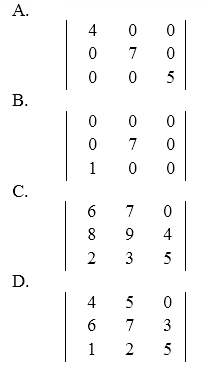195. Matrix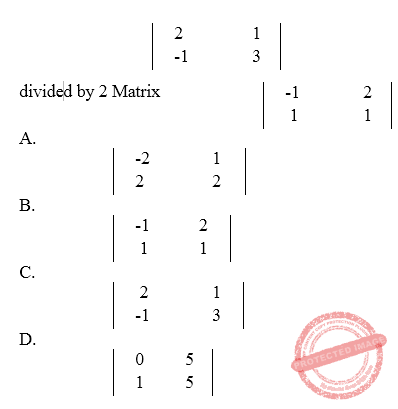196. Elements of matrix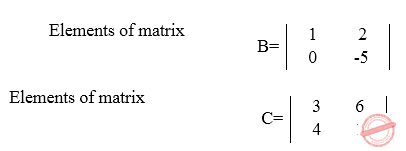Find the elements of the product of the two matrices, matrix BC.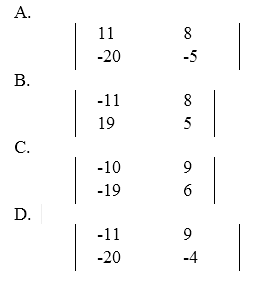197. Transpose the matrix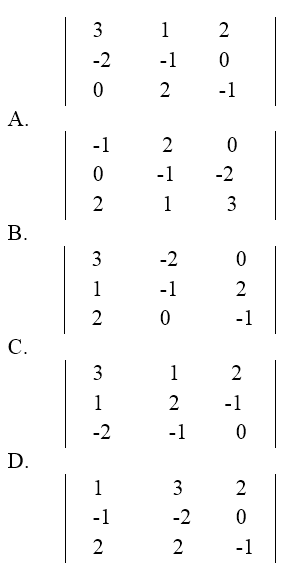198. Determine the inverse matrix of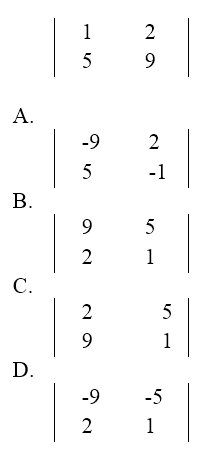199. k divided by [ (s square) + (k square) ] is the inverse laplace transform of:

• A. cos kt
• B. sin kt
• C. (e exponent kt)
• D. 1.0

200. The laplace transform of cos wt is

• A. s / [(s square) + (w square) ]
• B. w / [ (s square) + (w square) ]
• C. w / (s + w)
• D. s / (s + w)

### Online Questions and Answers in Calculus Series

Following is the list of multiple choice questions in this brand new series:

Calculus MCQs
PART 1: MCQs from Number 1 – 50                        Answer key: PART I
PART 2: MCQs from Number 51 – 100                        Answer key: PART II
PART 3: MCQs from Number 101 – 150                        Answer key: PART III
PART 4: MCQs from Number 151 – 200                        Answer key: PART IV
Help Me Makes a Difference!

 P inoyBIX educates thousands of reviewers/students a day in preparation for their board examinations. Also provides professionals with materials for their lectures and practice exams. Help me go forward with the same spirit. “Will you make a small \$5 gift today?” Option 1 : \$1 USD Option 2 : \$3 USD Option 3 : \$5 USD Option 4 : \$10 USD Option 5 : \$25 USD Option 6 : \$50 USD Option 7 : \$100 USD Option 8 : Other Amount© 2014 PinoyBIX Engineering. © 2019 All Rights Reserved | How to Donate? |#### GEAS Solution

Dynamics problem Economics problem Physics problem Statics problem Strength problem Thermodynamics problem

#### Questions and Answers in GEAS

Engineering Economics Engineering Laws and Ethics Engineering Management Engineering Materials Engineering Mechanics General Chemistry Physics Strength of Materials Thermodynamics
Consider Simple Act of Caring!: LIKE MY FB PAGE

Our app is now available on Google Play, Pinoybix Elex